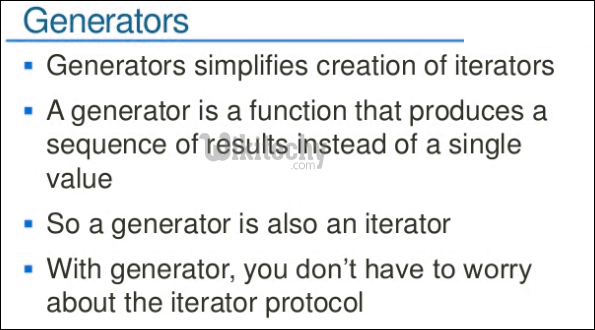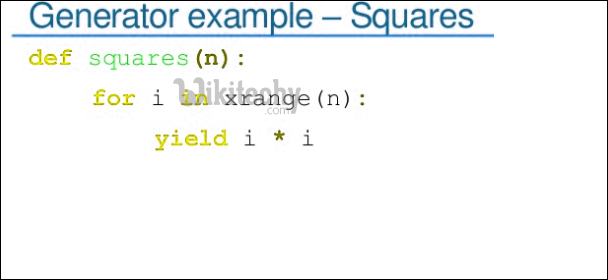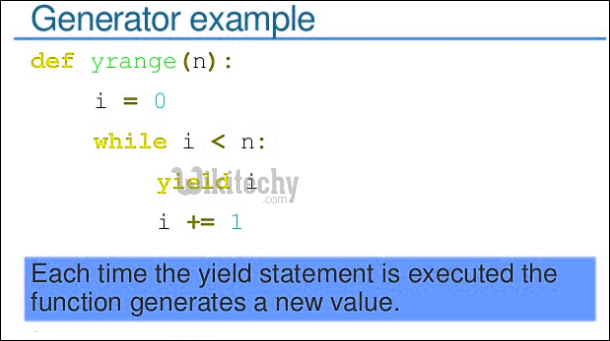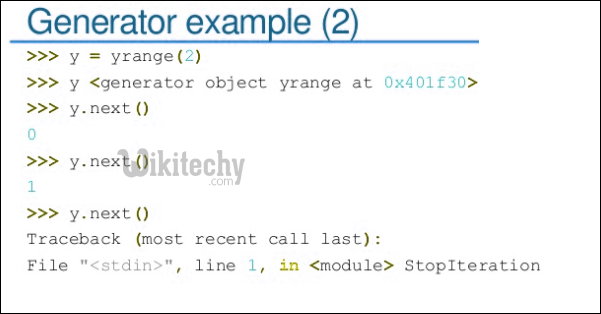# python tutorial - Python Generator | Generators in Python - learn python - python programming

There are two terms involved when we discuss generators.1. Generator-Function : A generator-function is defined like a normal function, but whenever it needs to generate a value, it does so with the yield keyword rather than return. If the body of a def contains yield, the function automatically becomes a generator function.

python - Sample - python code :

python programming - Output :

```1
2
3```
1. Generator-Object : Generator functions return a generator object. Generator objects are used either by calling the next method on the generator object or using the generator object in a “for in” loop (as shown in the above program).python - Sample - python code :

python programming - Output :

```1
2
3```

So a generator function returns an generator object that is iterable, i.e., can be used as an Iterators.As another example, below is a generator for Fibonacci Numbers.python - Sample - python code :

python programming - Output :

```0
1
1
2
3

Using for in loop
0
1
1
2
3```

Applications : Suppose we to create a stream of Fibonacci numbers, adopting the generator approach makes it trivial; we just have to call next(x) to get the next Fibonacci number without bothering about where or when the stream of numbers ends.
A more practical type of stream processing is handling large data files such as log files. Generators provide a space efficient method for such data processing as only parts of the file are handled at one given point in time. We can also use Iterators for these purposes, but Generator provides a quick way (We don’t need to write __next__ and __iter__ methods here).

Wikitechy tutorial site provides you all the learn python , online python programming course python programming class online , python coding for dummies , python programming classes online , online training python , online python training , python programming online course , python training classes